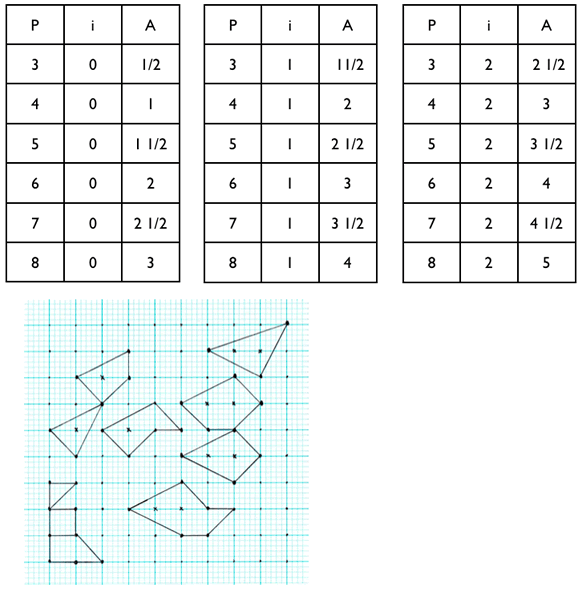#### You may also like### Diophantine N-tuples

Can you explain why a sequence of operations always gives you perfect squares?### DOTS Division

Take any pair of two digit numbers x=ab and y=cd where, without loss of generality, ab > cd . Form two 4 digit numbers r=abcd and s=cdab and calculate: {r^2 - s^2} /{x^2 - y^2}.### Sixational

The nth term of a sequence is given by the formula n^3 + 11n . Find the first four terms of the sequence given by this formula and the first term of the sequence which is bigger than one million. Prove that all terms of the sequence are divisible by 6.

# Pick's Theorem

##### Age 14 to 16Challenge Level

Yun from Garden Internation School thought that

The same number of dots on the perimeters and on the inside result in the same areas.
Red square: $(4, 0)$ $A=1$
Orange parellelogram: $(4, 0)$ $A=1$

Lily from Garden International School noticed that

as the sum of the perimeter increases the sum of the area increase by ½

Here are her results.

Kathryn, also from Garden International School, noticed two relationships:

As the number of dots on the shape's perimeter increases by one, the area increases by half.
As the number of internal dots increases by one, the area also increases by one.

Here are her results.Nadia from Melbourn Village College, Yun from Garden Internation School, Simeran and Aaron from Woodfield Junior School, and Kahlia from Merici College all discovered that:

When
$A =$ the area of the shape,
$p =$ the number of dots on the perimetre and
$i =$ the number of dots inside the shape,

the area is equal to half of $p$, added to $i$, minus $1$

$A = p/2 + i - 1$

This solution of Andrei's from School 205 Bucharest, gives a very useful algebraic view of the problem. He thought these were useful questions to be asking at the start:

How many different shapes can I draw of the form $(4,0)$?
Do they all have the same area? Can I see why?

Look at other shapes of the form $(n,0)$. What do these shapes have in common?
What about shapes of the form $(3,n)$?

By breaking the problem down in this way patterns emerged that gave hints about what was happening.

I took a look at the figures given in the problem, and I calculated the area $A$, the number of lattice points on the perimeter $p$ and the number of interior lattice points $i$. I found the table below:

#### i

Square
1
4
0
Triangle 1
3/2
3
1
Hexagon
6
6
4
Triangle 2
3/2
5
0
Parallelogram
1
4
0

I started from the idea that if any relation exists between $A, p$ and $i$, then there must be a linear relationship. Let $x$ be the coefficient of $A, y$ be the coefficient of $p$, and $z$ be the coefficient of $i$; $t$ is the free term. So:

$xA + yp + zi + t = 0$

Replacing the values corresponding to the first four rows (because the last is identical with the first) I found a system of $4$ equations with four unknowns:

$x + 4y + t = 0$
$3/2x + 3y +z + t = 0$
$4x + 6y + 4z + t = 0$
$3/2x + 5y + t = 0$

Except the $(0,0,0,0)$ solution, I found the relation between $x, y, z$ and $t$ either of the type:

$x = -i, y = i/2, z = i, t = -i,$
or:
$x = i, y = -i/2, z = -i, t = i.$

The last two solutions imply that the relation between $A, p$ and $i$ must be:

$-A + p/2 + i -1 = 0$

or:

$A = p/2 + i -1$

This is not a demonstration, from what I have found I can't be sure it works always. But I have looked on the web, and I found some derivations of Pick's theorem, that looks as I found it. One is at Geoboards in the classroom and another is at Cut the Knot

This is a method of calculating the area of any polygon on a geoboard quickly and easily. The theorem has been found by Georg Alexander Pick, born in 1859 in Vienna, and was first published in 1899.